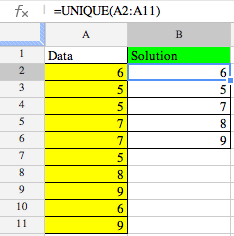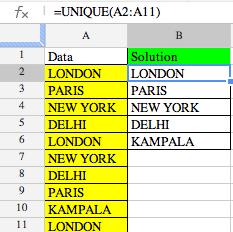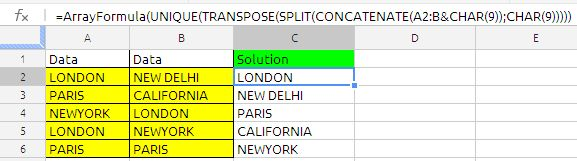# UNIQUE FUNCTION

UNIQUE

Unique function is unique to google docs spreadsheets, the Returns only the unique rows in the source array, duplicates will be ignored

The syntax of the function is as follows:

UNIQUE(SourceArray)

SourceArray:  range of cells, number, date or string, data has to be of the same type, it can also be array formula results.

Note:

• The output of the unique function will in the same order as that of SourceArray.

• unique values will give only for vertical array only

• to get unique values from a horizontal array we have to use the transpose function inside the unique function.

Example 1:the data is cell A2:A11 to extract the unique value from the formula used in cell B2 is

=unique(A2:A1)

Example 2:we can extract the unique values from column A&B the formula in cell C2 is

=ArrayFormula(UNIQUE(TRANSPOSE(SPLIT(CONCATENATE(A2:B&CHAR(9));CHAR(9)))))

the explanation to the above formula at the following link

https://anandexcels.wordpress.com/2012/02/14/extracting-unique-records-from-two-columns/